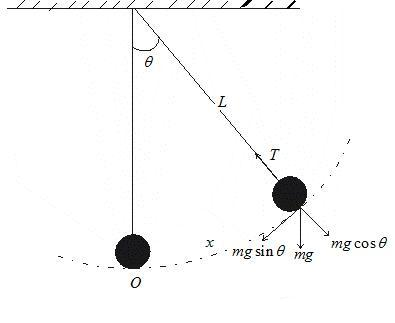# Simple Harmonic Motion calculator

Simple PendulumSimple Pendulum Length(m): Acceleration due to gravity(m/s2): Timeperiod(N):

Note
• Enter the values of the two known variables in the text boxes
• Leave the text box empty for the variable you want to solve for
• Click on the calculate button.

Example of Few questions where you can use this formula
• An simple pendulum as lenght .1 m and acceleration due to gravity at that place is 10 m/s2.Find the timeperiod of small oscillation?
• An simple pendulum as lenght .2 m and Timeperiod 10 sec.Find the acceleration due to gravity at that place?

Latest Articles
Synthetic Fibres and Plastics Class 8 Practice questions

Class 8 science chapter 5 extra questions and Answers

Mass Calculator

3 Fraction calculator

Garbage in Garbage out Extra Questions7